双边滤波器的定义
双边滤波器（Bilateral filter）为使图像平滑化的非线性滤波器，它除了使用像素之间几何上的靠近程度之外，还多考虑了像素之间的灰度差异， 使得双边滤波器能够有效的将图像上的噪声去除，同时保存图像上的边缘信息。
双边滤波器的好处是可以做边缘保护（edge preserving），一般用高斯滤波、均值滤波等滤波器去降噪，会较明显地模糊边缘，对于高频细节的保护效果并不明显。双边滤波器顾名思义比高斯滤波多了一个高斯方差sigma-d，它是基于空间分布的高斯滤波函数，所以在边缘附近，离的较远的像素不会太多影响到边缘上的像素值，这样就保证了边缘附近像素值的保存。但是由于保存了过多的高频信息，对于彩色图像里的高频噪声，双边滤波器不能够干净的滤掉，只能够对于低频信息进行较好的滤波。
双边滤波器的原理
空间距离：指的是邻域内某点与中心点的欧式距离。空间域高斯函数其数学形式为（这就是高斯滤波核）：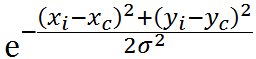其中（xi,yi）为邻域内某点位置，（xc,yc）为中心点的位置，sigma为空间域标准差。
灰度距离：指的是邻域内某点灰度与中心点灰度的差的绝对值。值域高斯函数其数学形式为：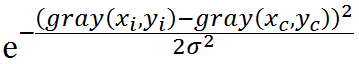其中gray(xi,yi)为邻域内某点灰度值，gray(xc,yc)为中心点灰度值，sigma为值域标准差。
对于高斯滤波，仅用空间距离的权值系数核与图像卷积后，确定中心点的灰度值。即认为离中心点越近的点，其权重系数越大。双边滤波中加入了对灰度信息的权重，即在邻域内，灰度值越接近中心点灰度值的点的权重更大，灰度值相差大的点权重越小。所以掩膜最终权重大小，则由空间域高斯核函数和值域高斯核函数共同确定。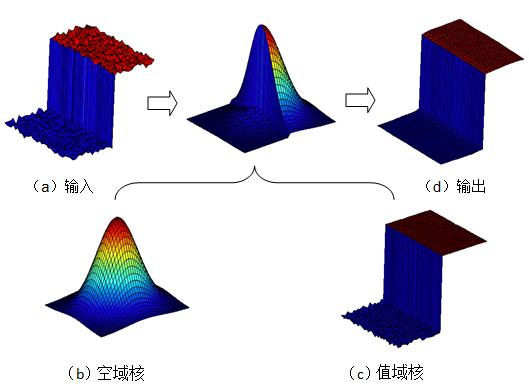双边滤波器的MATLAB代码实现
bftGrayImage.m文件
function J=bftGrayImage(I,G,sigma_d,sigma_r,filterRadius)
J=zeros(size(I));
[xx,yy]=meshgrid(x,y);
spatialCoeffs=exp(- (xx.^2+yy.^2)/(2*sigma_d^2));
[rows,cols]=size(I);
centerValue=G(y,x);
roidif=roi-centerValue;
tonalWeight =exp(- roidif.^2/(2*sigma_r^2));
F=(tonalWeight.*spatialCoeffs);
k=sum(F(:));
weight=F.*roi;
J(y,x)=sum(weight(:))/k;
end
end
J=mat2gray(J);
end
main.m文件调用：

g = rgb2gray(g);
G=g;%guidance image
sigma_d=2;
sigma_r=0.3;
filterSize=double(uint8(3*sigma_d)*2+1);

%figure;imshow([g,J]);title('input,output');
figure;
imshow(g,[]);
title('input');
figure;
imshow(J,[]);
title('output');
σ的意义及选取 1）空间域sigma-d选取：
sigma-d越大，图像越平滑，趋于无穷大时，每个权重都一样，类似均值滤波；
sigma-d越小，中心点权重越大，周围点权重越小，对图像的滤波作用越小，趋于零时，输出等同于原图。
2）值域sigma-r选取：
Sigma-r越大，边缘越模糊，极限情况为simga无穷大，值域系数近似相等（忽略常数时，将近为exp（0）= 1），与高斯模板（空间域模板）相乘后可认为等效于高斯滤波。
Sigma-r越小，边缘越清晰，极限情况为simga无限接近0，值域系数除了中心位置，其他近似为0（接近exp（-∞） =  0），与高斯模板（空间域模板）相乘进行滤波的结果等效于源图像。  
展开全文双边滤波
• 正是r函数的作用，使得边缘，即相距近但差异大的像素点的特性得以保留。 / ** @brief将双边过滤器应用于图像。 可以在保持边缘相当清晰的同时很好地减少不必要的噪音。但是，与大多数过滤器相比，它非常慢。 _Sigma ...


文章目录
一. 线性滤波1.1. 方框滤波demo
1.2. 均值滤波demo
1.3. 高斯滤波demo

二. 非线性滤波2.1. 中值滤波demo
2.2. 双边滤波demo

结构体参考

一. 线性滤波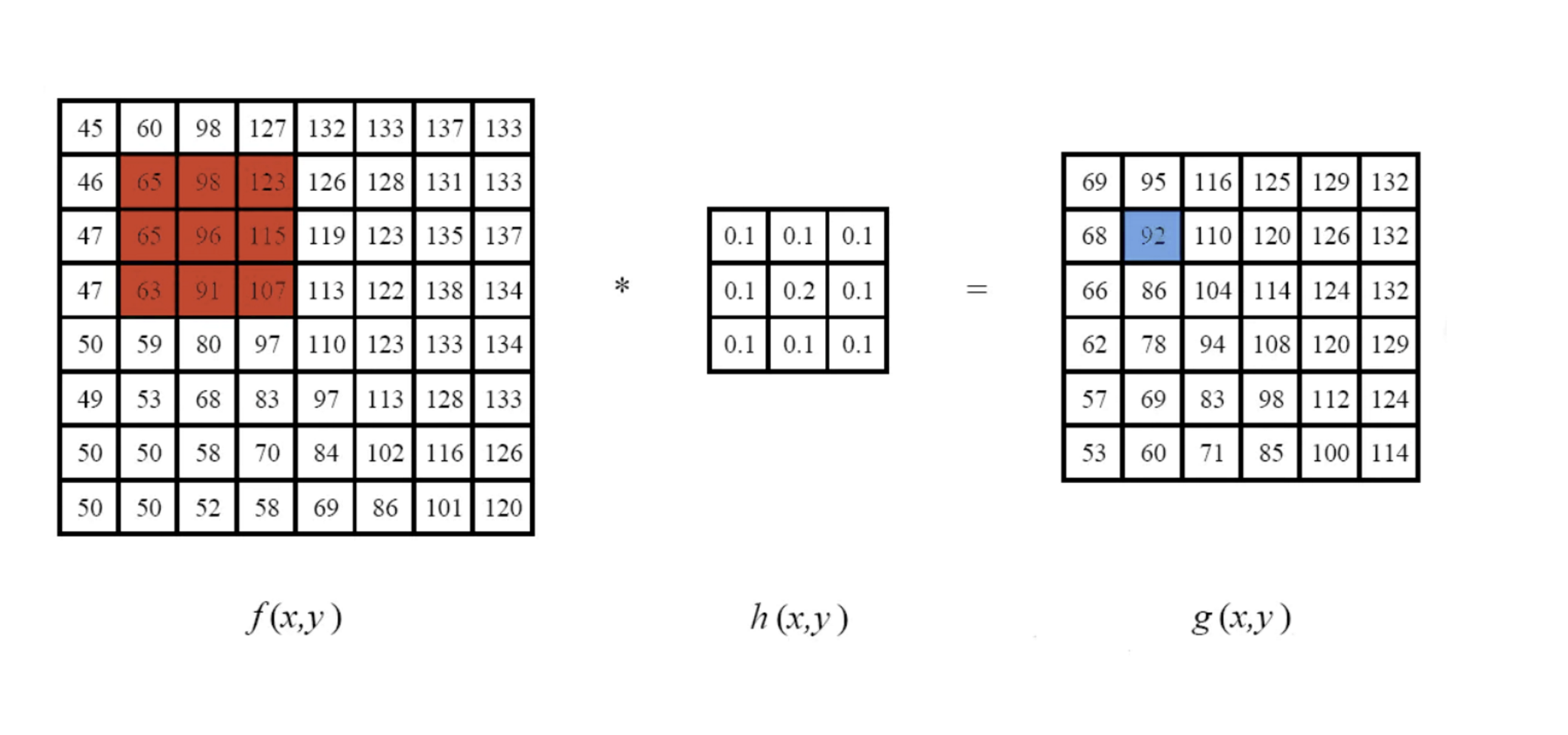1.1. 方框滤波
方框滤波是所有滤波器中最简单的一种滤波方式。每一个输出像素的是内核邻域像素值的平均值得到。 通用的滤波kernel如下：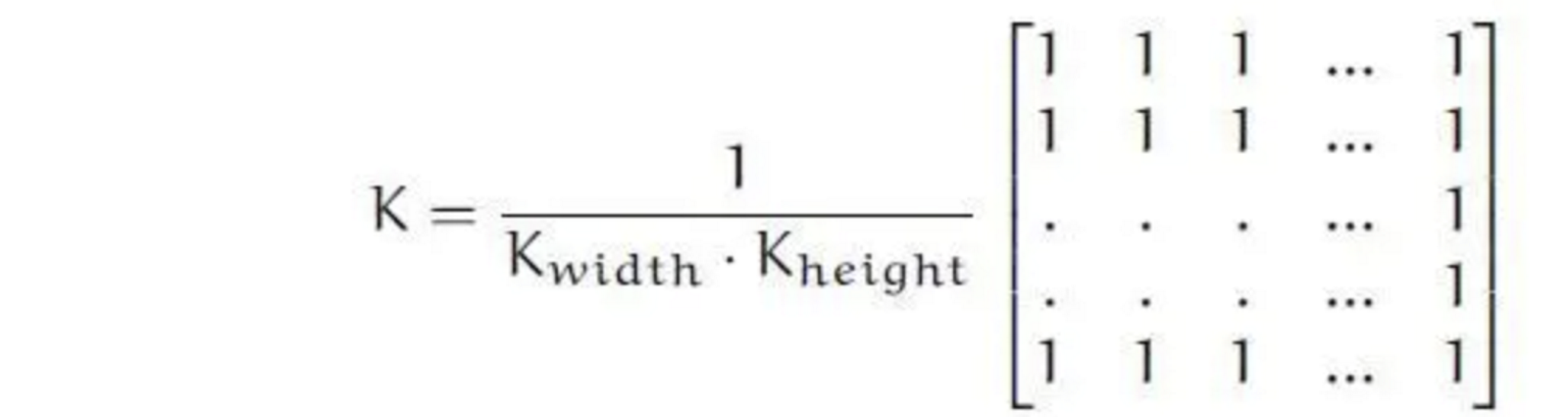这里是一个长宽分别为Kwidth和Kheight的窗口函数，在此区域内邻域中像素值叠加求平均即可求出位于kernel中心点像素的像素值。
/ ** @brief使用框过滤器模糊图像。
该函数使用内核对图像进行平滑处理：
未归一化的框式滤波器可用于计算每个像素邻域的各种积分特征，
例如图像导数的协方差矩阵（用于密集光流算法等）。
如果需要在可变大小的窗口上计算像素总和，请使用#integral。

@param src输入图像。
@param dst输出图像的大小和类型与src相同。
@param ddepth输出图像深度（使用src.depth（）时为-1）。
@param ksize模糊内核的大小。一般Size(w,h)来表示内核的大小。
@param 锚点（即被平滑的那个点）；默认值Point（-1，-1）表示锚点位于内核中心。
@param normalize标志，指定是否通过内核区域对其进行规范化。
@param borderType用于推断图像外部像素的边框模式，请参阅#BorderTypes
* /

CV_EXPORTS_W void boxFilter( InputArray src, OutputArray dst, int ddepth,
Size ksize, Point anchor = Point(-1,-1),
bool normalize = true,
int borderType = BORDER_DEFAULT );

boxFilter（）函数方框滤波所用的核为：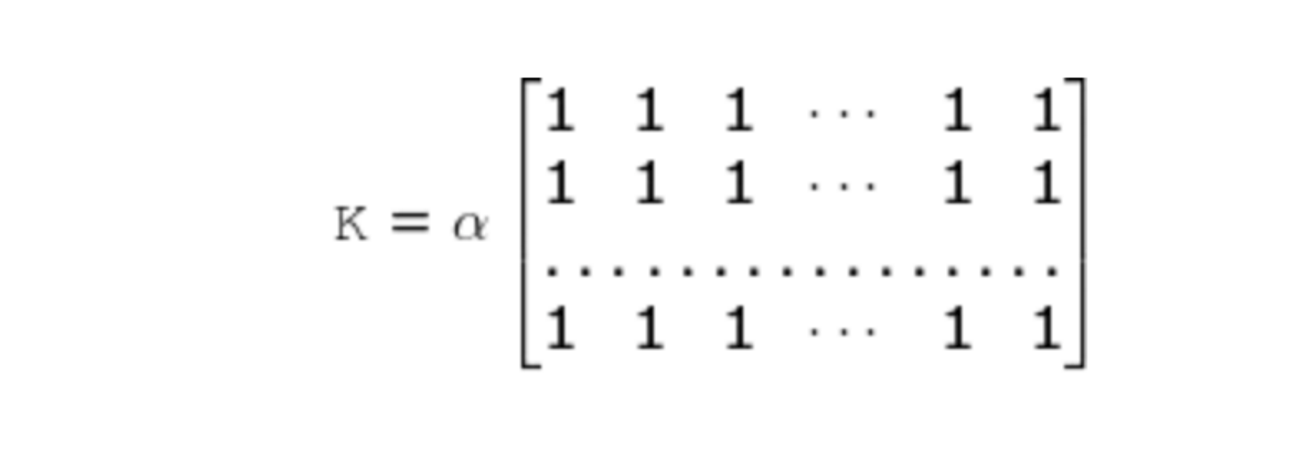其中：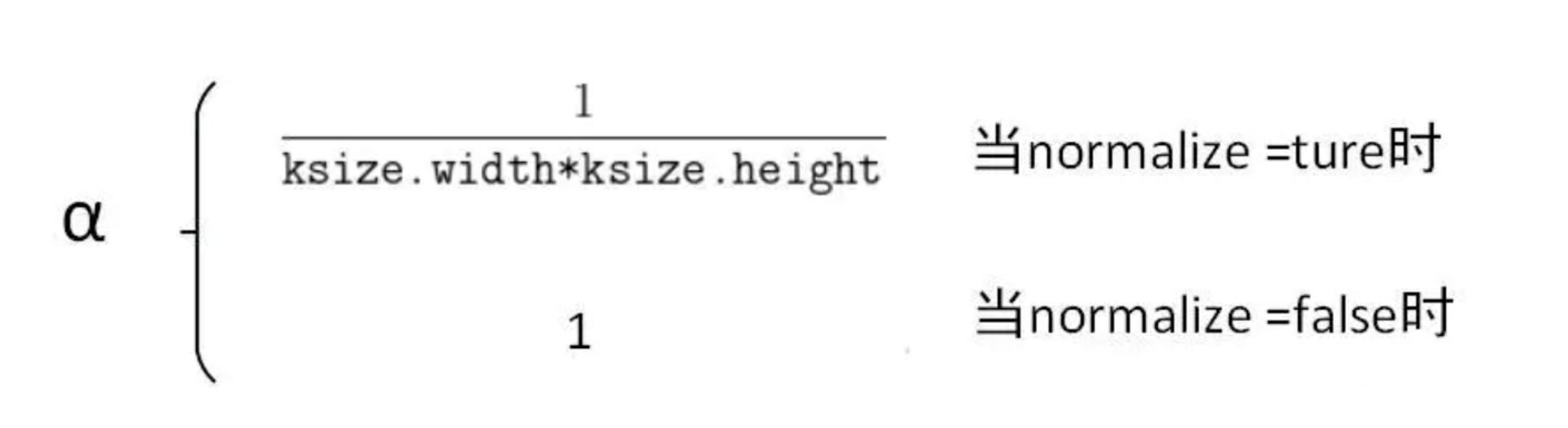当normalize=true的时候，方框滤波就变成了下面要说的的均值滤波。
demo
int MPT_test_boxFilter()
{
cv::Mat dst1, dst2, dst3, dst4;
cv::boxFilter(src, dst1, -1, cv::Size(5, 5), cv::Point(-1, -1), true);
cv::namedWindow("src", 0);
cv::imshow("src", src);
cv::namedWindow("方框滤波", 0);
cv::imshow("方框滤波", dst1);
cv::waitKey(0);
return 0;
}1.2. 均值滤波
均值滤波的原理非常简单，就是输出图像的每一个像素是核窗口内输入图像对应像素的像素的平均值( 所有像素加权系数相等)，其实说白了它就是归一化后的方框滤波。
但是均值滤波本身存在着固有的缺陷，即它不能很好地保护图像细节，在图像去噪的同时也破坏了图像的细节部分，从而使图像变得模糊，不能很好地去除噪声点。
/ ** @brief使用标准化框过滤器模糊图像。

该函数使用内核对图像进行平滑处理：
@param src输入图像； 它可以具有任意数量的通道，这些通道是独立处理的，但是深度应为CV_8U，CV_16U，CV_16S，CV_32F或CV_64F。
@param dst输出图像的大小和类型与src相同。
@param ksize模糊内核大小。
@param锚点； 默认值Point（-1，-1）表示锚点位于内核中央。
@param borderType用于推断图像外部像素的边框模式，请参阅#BorderTypes
*/
CV_EXPORTS_W void blur( InputArray src, OutputArray dst,
Size ksize, Point anchor = Point(-1,-1),
int borderType = BORDER_DEFAULT );

demo
//均值滤波
int MPT_test_blur() {
cv::Mat dst;
cv::blur(src, dst, cv::Size(5, 5));
cv::namedWindow("src", 0);
cv::imshow("src", src);

cv::namedWindow("均值滤波", 0);
cv::imshow("均值滤波", dst);
cv::waitKey(0);
return 0;
}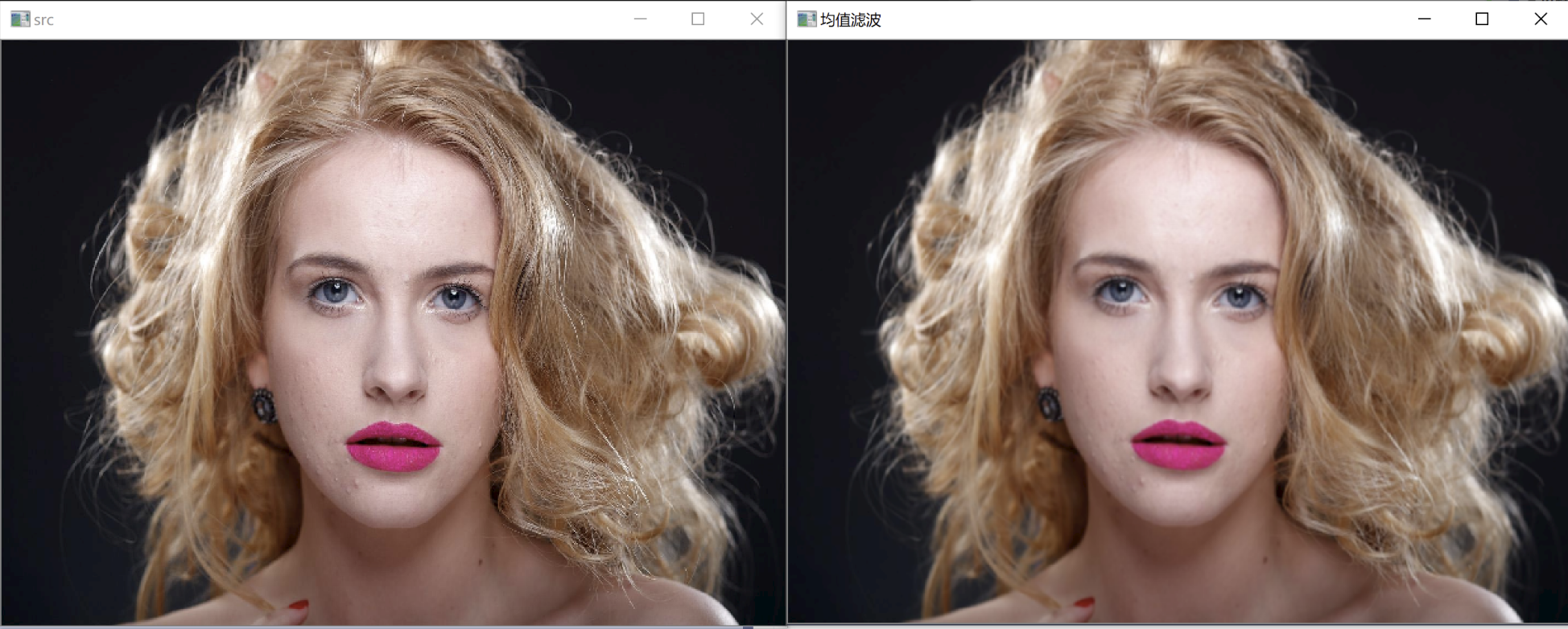1.3. 高斯滤波
图像的高斯模糊过程就是图像与服从二维正态分布的卷积核做卷积。由于正态分布又叫作高斯分布，所以这项技术就叫作高斯模糊。 图像与圆形卷积核做卷积将会生成更加精确的焦外成像效果。由于高斯函数的傅立叶变换是另外一个高斯函数，所以高斯模糊对于图像来说就是一个低通滤波操作。 高斯滤波器是一类根据高斯函数的形状来选择权值的线性平滑滤波器。高斯平滑滤波器对于抑制服从正态分布的噪声非常有效。
一维零均值高斯函数为：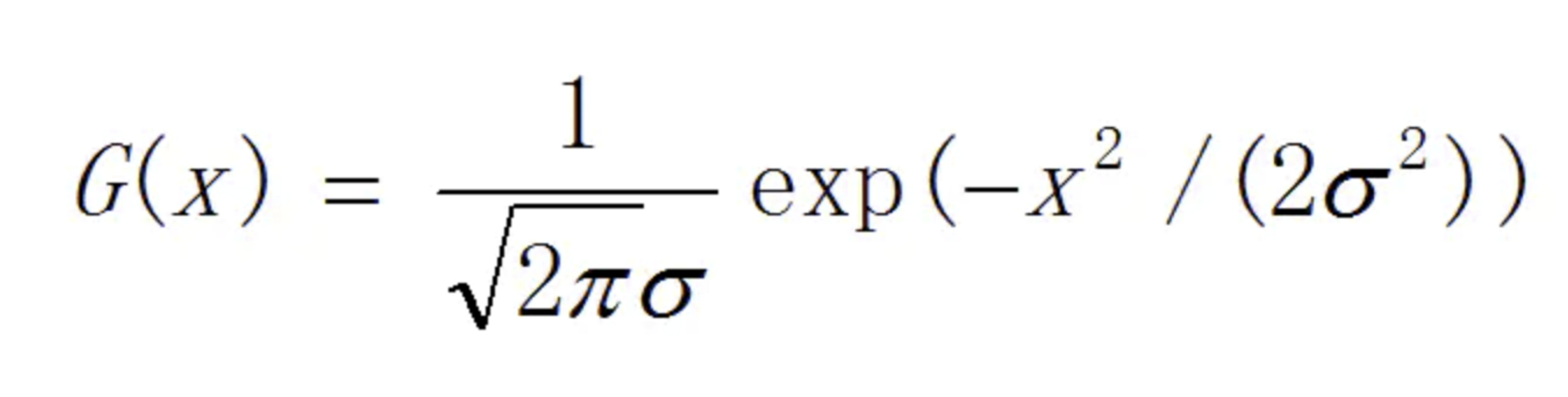其中，高斯分布参数σ决定了高斯函数的宽度。
对于二维图像来说，常用二维零均值离散高斯函数作平滑滤波器。 二维高斯函数为：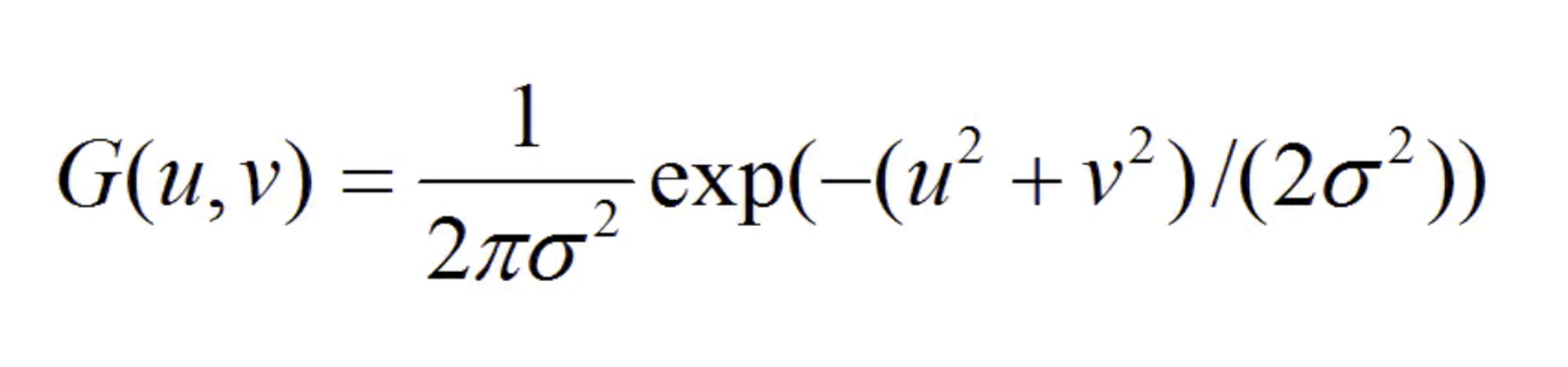/ ** @brief使用高斯滤镜模糊图像。

该函数将源图像与指定的高斯内核进行卷积。就地过滤是
支持的。

@param src输入图像；图像可以具有任意数量的经过处理的通道
但深度应为CV_8U，CV_16U，CV_16S，CV_32F或CV_64F。
@param dst输出图像的大小和类型与src相同。
@param ksize高斯内核大小。 ksize.width和ksize.height可以不同，但它们都必须为正数和奇数。或者，它们可以为零，然后根据sigma计算得出。
@param sigmaX X方向上的高斯核标准偏差。
@param sigmaY Y方向上的高斯核标准差；如果sigmaY为零，则将其设置为等于sigmaX；如果两个sigmas为零，则分别从ksize.width和ksize.height计算得出（有关详细信息，请参见#getGaussianKernel）；为了完全控制结果，而不考虑将来可能对所有这些语义的修改，建议指定所有ksize，sigmaX和sigmaY。
@param borderType像素外推方法，请参见#BorderTypes
*/

CV_EXPORTS_W void GaussianBlur( InputArray src, OutputArray dst, Size ksize,
double sigmaX, double sigmaY = 0,
int borderType = BORDER_DEFAULT );

demo
//高斯滤波
int MPT_test_GaussianBlur() {
cv::Mat dst;
cv::GaussianBlur(src, dst, cv::Size(5, 5), 0, 0);
cv::namedWindow("src", 0);
cv::imshow("src", src);

cv::namedWindow("高斯滤波", 0);
cv::imshow("高斯滤波", dst);
cv::waitKey(0);
return 0;
}二. 非线性滤波
非线性滤波器的原始数据与滤波结果是一种逻辑关系，即通过比较一定邻域内的灰度值大小来实现的。
2.1. 中值滤波
中值滤波原理：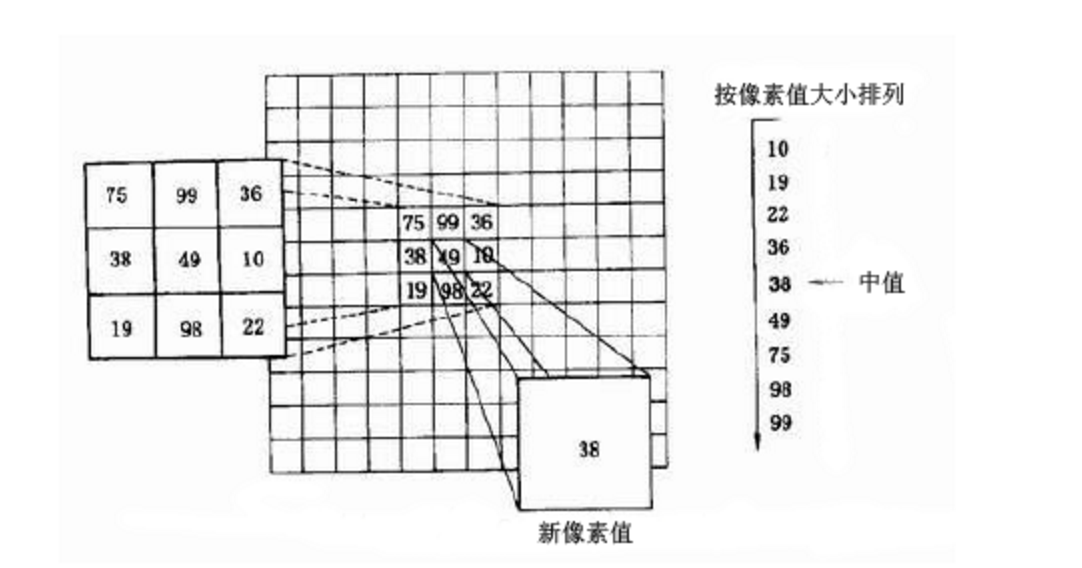简言之中值滤波就是把函数框（如图中的3 X 3）内的灰度值按顺序排列，然后中值取代函数框中心的灰度值。所以一般采用奇数点的邻域来计算中值，但如果像素点数为偶数，中值就取排序像素中间两点的平均值。
中值滤波在一定的条件下可以克服常见线性滤波器如方框滤波器、均值滤波等带来的图像细节模糊，而且对滤除脉冲干扰及图像扫描噪声非常有效，也常用于保护边缘信息, 保存边缘的特性使它在不希望出现边缘模糊的场合也很有用，是非常经典的平滑噪声处理方法。
但是中值滤波的缺点也很明显，因为要进行排序操作，所以处理的时间长，是均值滤波的5倍以上。
/ ** @brief使用中值滤镜模糊图像。
@note中值过滤器内部使用#BORDER_REPLICATE来处理边框像素，请参阅#BorderTypes

@param src输入1、3或4通道图像;当ksize为3或5时，
图像深度应为CV_8U，CV_16U或CV_32F，对于较大的光圈，只能为CV_8U。
@param dst目标数组，其大小和类型与src相同。
@param ksize孔径线性大小； 它必须是奇数且大于1，例如：3、5、7 ...
*/

CV_EXPORTS_W void medianBlur( InputArray src, OutputArray dst, int ksize );

demo
//中值滤波
int MPT_test_medianBlur() {
cv::Mat dst;
cv::medianBlur(src, dst, 9);
cv::namedWindow("src", 0);
cv::imshow("src", src);

cv::namedWindow("中值滤波", 0);
cv::imshow("中值滤波", dst);
cv::waitKey(0);
return 0;
}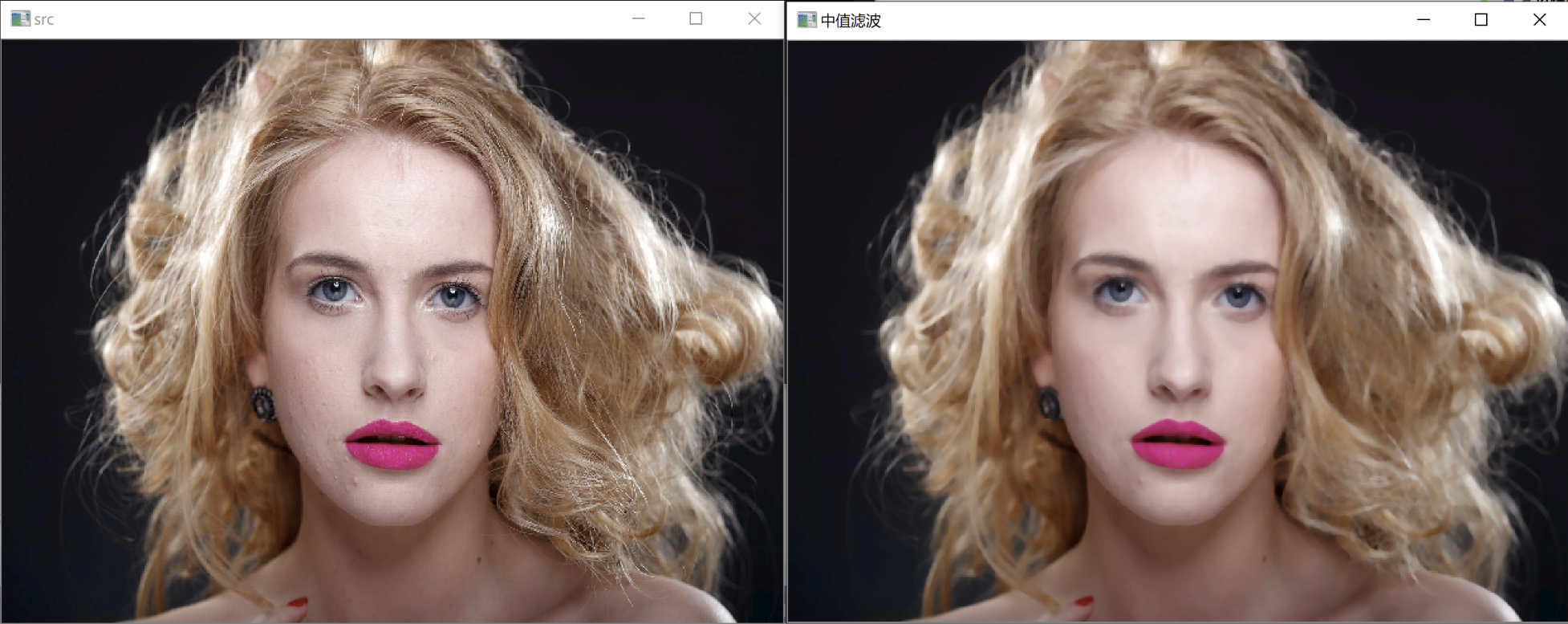2.2. 双边滤波
双边滤波（Bilateral filter）是一种非线性的滤波方法，是结合图像的空间邻近度和像素值相似度的一种折衷处理，同时考虑空域信息和灰度相似性，达到保边去噪的目的。具有简单、非迭代、局部的特点。
双边滤波器的好处是可以做边缘保存（edge preserving），一般用高斯滤波去降噪，会较明显地模糊边缘，对于高频细节的保护效果并不明显。双边滤波器顾名思义比高斯滤波多了一个高斯方差sigma－d，它是基于空间分布的高斯滤波函数，所以在边缘附近，离的较远的像素不会太多影响到边缘上的像素值，这样就保证了边缘附近像素值的保存。但是由于保存了过多的高频信息，对于彩色图像里的高频噪声，双边滤波器不能够干净的滤掉，只能够对于低频信息进行较好的滤波。
下图是双边滤波的原理示意图：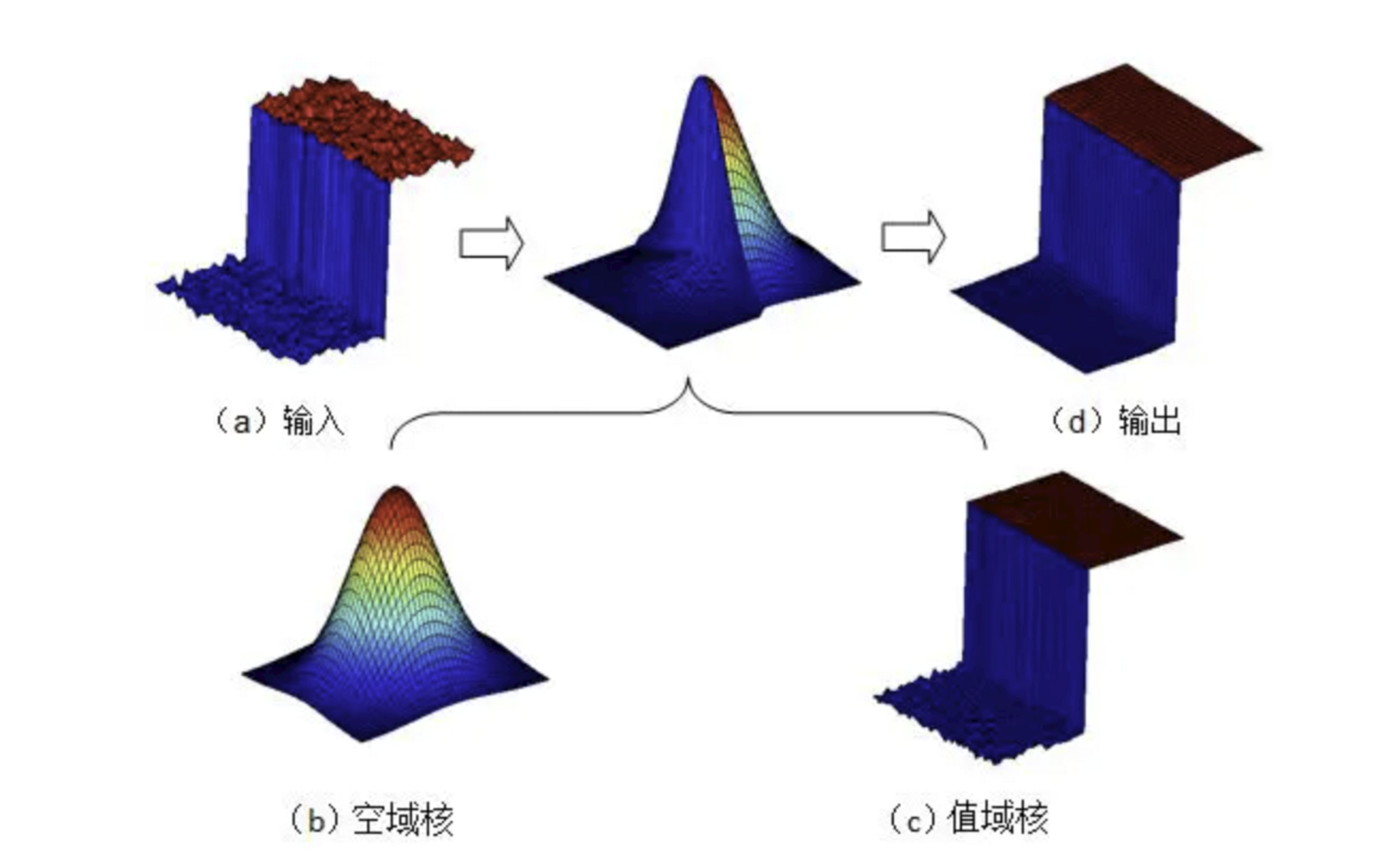在双边滤波器中，输出像素的值依赖于邻域像素值的加权值组合：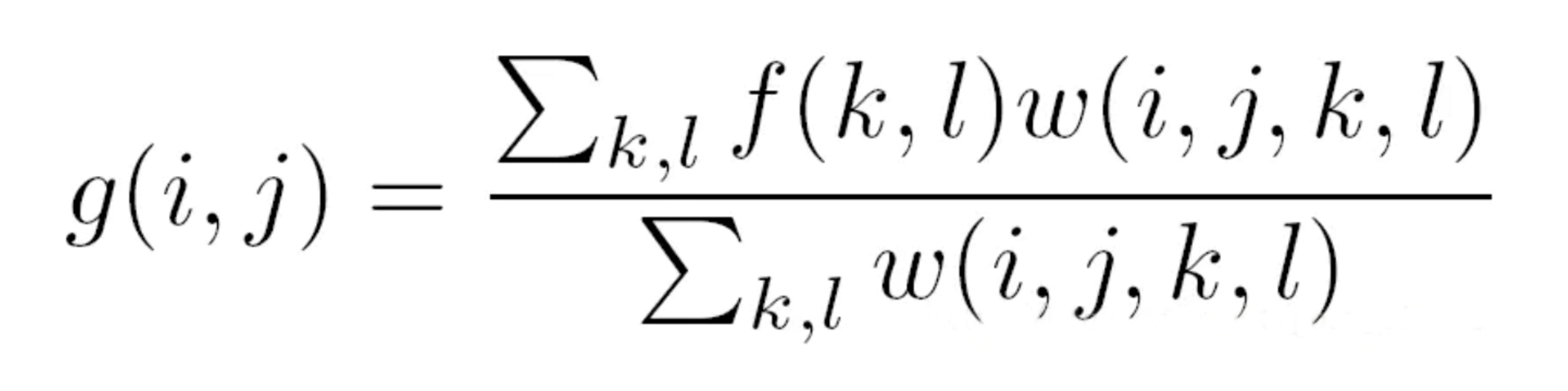而加权系数w(i,j,k,l)取决于空域核和值域核的乘积。 (i,j)，(k,l)分别指两个像素点的坐标。 其中空域核表示如下（如图）：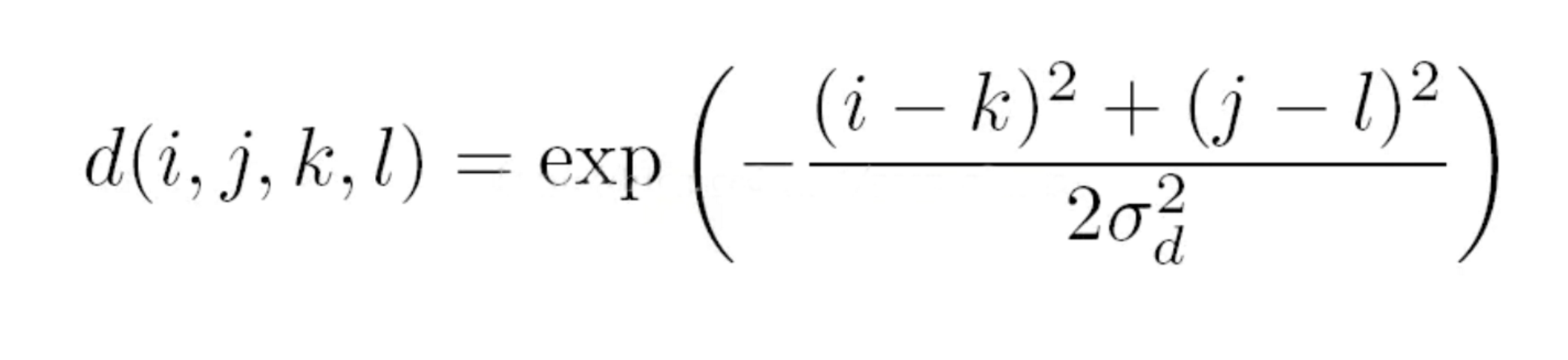值域核表示为：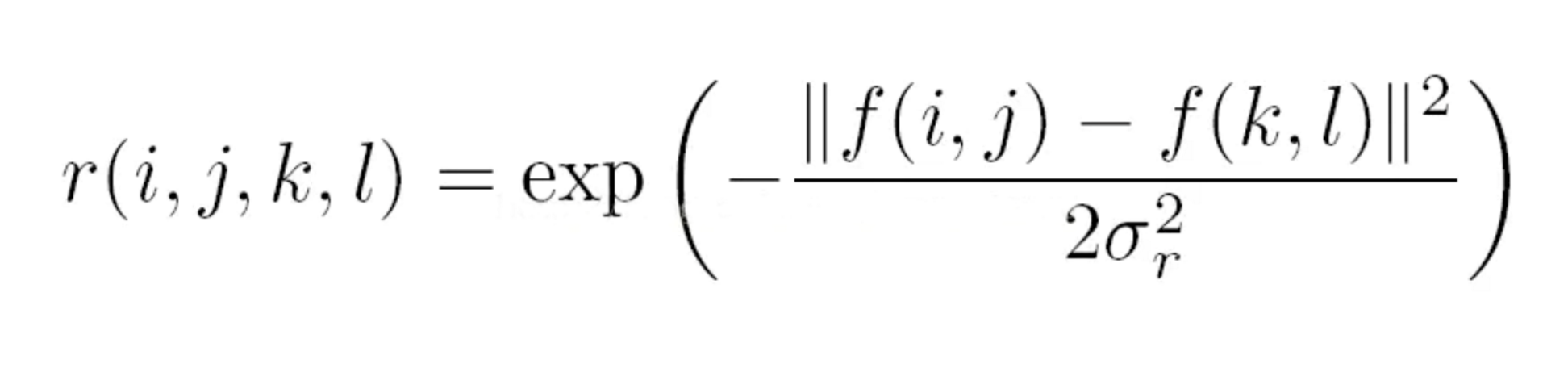两者相乘后，就会产生依赖于数据的双边滤波权重函数：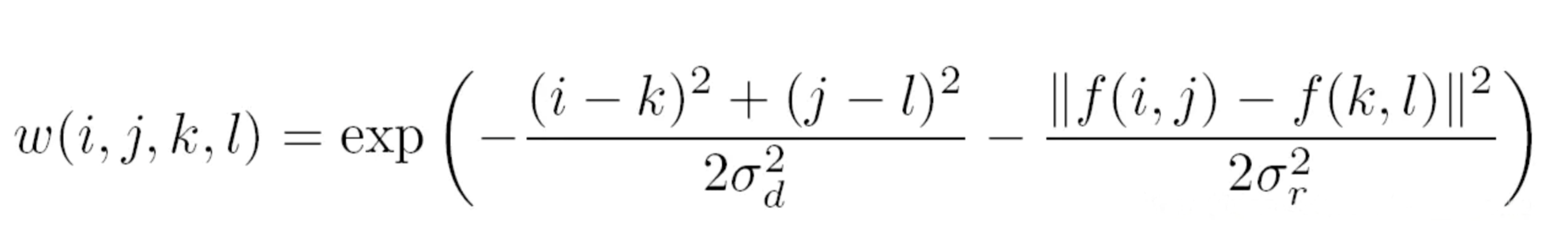d函数是根据像素距离选择权重，距离越近权重越大，这一点和方框滤波，高斯滤波方式相同。而r函数则是根据像素的差异来分配权值。如果两个像素值越接近，即使相距较远，也比差异大而距离近的像素点权重大。正是r函数的作用，使得边缘，即相距近但差异大的像素点的特性得以保留。
/ ** @brief将双边过滤器应用于图像。
可以在保持边缘相当清晰的同时很好地减少不必要的噪音。但是，与大多数过滤器相比，它非常慢。

_Sigma values_：为简单起见，您可以将2个sigma值设置为相同。如果它们很小（<10），则滤镜效果不大；
而如果它们很大（> 150），它们将具有非常大的效果。效果强，使图像看起来“卡通化”。

_Filter size_：大型滤镜（d > 5）非常慢，因此建议实时使用d = 5
应用程序，对于需要重噪声过滤的脱机应用程序，d = 9。

@param src  源8位或浮点，1通道或3通道图像。
@param dst  与src大小和类型相同的目标映像。
@param d    滤波期间使用的每个像素邻域的直径。如果它不是正值，
则从sigmaSpace计算得出。
@param sigmaColor 在色彩空间中过滤sigma。
这个参数的值越大，就表明该像素邻域内有更宽广的颜色会被混合到一起，产生较大的半相等颜色区域
@param sigmaSpace 在坐标空间中过滤sigma。
该参数的值越大，表示越远的像素就会相互影响，
只要它们的颜色足够接近即可（请参见sigmaColor）。
当d > 0时，它指定邻域大小，而不考虑sigmaSpace。
否则，d为与sigmaSpace成比例。
@param borderType 用于推断图像外部像素的边框模式，请参阅#BorderTypes

*/

CV_EXPORTS_W void bilateralFilter( InputArray src, OutputArray dst, int d,
double sigmaColor, double sigmaSpace,
int borderType = BORDER_DEFAULT );


demo
//双边滤波
int MPT_test_bilateralFilter() {
cv::Mat dst;
cv::bilateralFilter(src, dst, 25, 10.0, 25);
cv::namedWindow("src", 0);
cv::imshow("src", src);
cv::namedWindow("双边滤波", 0);
cv::imshow("双边滤波", dst);
cv::waitKey(0);
return 0;
}结构体参考
enum BorderTypes {
BORDER_CONSTANT    = 0, //!< iiiiii|abcdefgh|iiiiiii  with some specified i
BORDER_REPLICATE   = 1, //!< aaaaaa|abcdefgh|hhhhhhh
BORDER_REFLECT     = 2, //!< fedcba|abcdefgh|hgfedcb
BORDER_WRAP        = 3, //!< cdefgh|abcdefgh|abcdefg
BORDER_REFLECT_101 = 4, //!< gfedcb|abcdefgh|gfedcba
BORDER_TRANSPARENT = 5, //!< uvwxyz|abcdefgh|ijklmno

BORDER_REFLECT101  = BORDER_REFLECT_101, //!< same as BORDER_REFLECT_101
BORDER_DEFAULT     = BORDER_REFLECT_101, //!< same as BORDER_REFLECT_101
BORDER_ISOLATED    = 16 //!< do not look outside of ROI
};

展开全文opencv 图像处理 滤波 高斯滤波 双边滤波
• temp = f_temp(i-r:i+r,j-r:j+r); % 一个局部块的像素值 w_pixel = exp( -( temp- img(i-r,j-r) ).^2/(2*b^2)); % 值域核 w = w_spatial .* w_pixel; s = temp.*w; res(i-r,j-r) = sum(s(:)) / sum(w(:)); ...
参考: https://blog.csdn.net/MoFMan/article/details/77482794 https://www.jianshu.com/p/8d11e26c9665 代码:https://blog.csdn.net/l_eop/article/details/81812277 高斯滤波:https://blog.csdn.net/nima1994/article/details/79776802
滤波
在介绍双边滤波以前,简要介绍一下滤波的过程.就像下面的动图所展示的,我们使用一个3×3的卷积核以步长为1对图像进行遍历,然后得到一幅新的经过滤波的图像.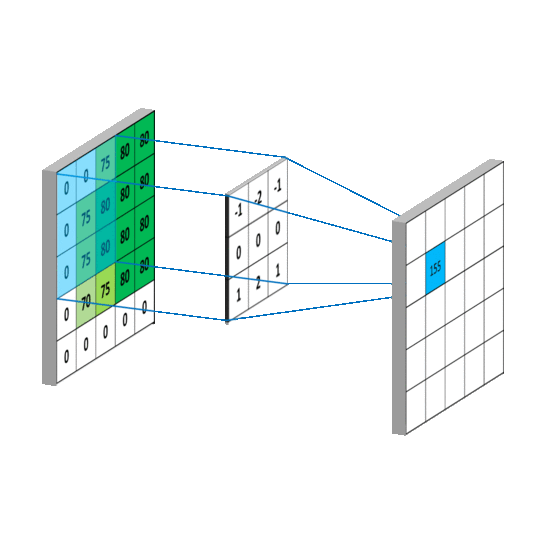其中每次滤波的时候只是在计算中心点的新的像素值.也就是说每次遍历移动一步其实只是在利用邻域(n×n)计算一个像素点的值,直到将图像中所有的点都卷积一次.那么卷积的结果就是,在3×3的邻域内,权重矩阵和图像块之间各对应位置相乘然后将这些乘积相加得到红色中心点的新的像素值.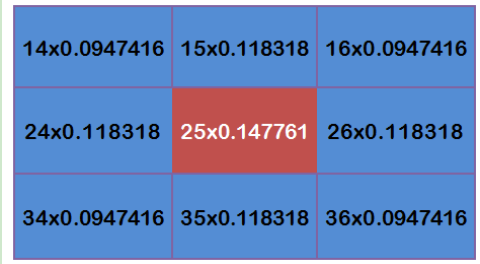双边滤波
双边滤波是一种非线性的方法,同时考虑到了图像的空域信息和灰度相似性,以达到保边去噪的目的.双边滤波通过空域矩阵和值域矩阵形成一个新的权重矩阵,其中空域矩阵用来模糊去噪；值域矩阵用来保护边缘. 在介绍相关公式之前,我们定义(i,j)为中心点坐标,(k,l)为以(i,j)点为中心的邻域S内的任意一点. 空域矩阵中点(k,l)到点(i,j)的空间距离定义为:

d

(

i

,

j

,

k

,

l

)

=

e

x

p

−

(

i

−

k

)

2

+

(

j

−

l

)

2

2

σ

d

2

(

1

)

d(i,j,k,l)=exp-\frac{(i-k)^2+(j-l)^2}{2σ_{d}^2} (1)

值得注意的一旦

σ

d

σ_{d}

确定了,那么空域矩阵中各点的值是始终不变的. 而值域矩阵定义为:

r

(

i

,

j

,

k

,

l

)

=

e

x

p

−

∣

∣

f

(

k

,

l

)

−

f

(

i

,

j

)

∣

∣

2

2

σ

r

2

(

2

)

r(i,j,k,l)=exp-\frac{||f(k,l)-f(i,j)||^2}{2σ_{r}^2} (2)

两者相乘后，就会产生依赖于数据的双边滤波权重矩阵w(i,j,k,l)：

w

(

i

,

j

,

k

,

l

)

=

e

x

p

−

(

i

−

k

)

2

+

(

j

−

l

)

2

2

σ

d

2

−

∣

∣

f

(

k

,

l

)

−

f

(

i

,

j

)

∣

∣

2

2

σ

r

2

(

3

)

w(i,j,k,l)=exp-\frac{(i-k)^2+(j-l)^2}{2σ_{d}^2} -\frac {||f(k,l)-f(i,j)||^2}{2σ_{r}^2} (3)

最后计算得到点(i,j)新的像素值g(i,j).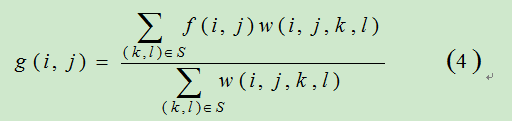d函数根据像素距离选择权重，距离越近权重越大,这一点和方框滤波,高斯滤波方式相同.而r函数则是根据像素值的差异来分配权值. 在平坦区域,像素差异较小,对应值域权重r(i,j,k,l)接近于1,此时空域权重d(i,j,k,l)起主要作用,相当于直接对此平坦区域进行高斯模糊.需要注意的是空域核是始终不变的,而值域核却因为区域的不同而不同. 边缘的特点就是相距近的点像素值差异大.那么滤波的同时是希望保留边缘的这种特性的.所以在边缘区域,因为像素之间差异较大,即f(k,l)-f(i,j)之间差值大,此时值域核权重变小,导致此处总权重值w(i,j,k,l)下降(w=r*d),当前像素(i,j)受到大差异点的影响就越小,从而保持了边缘的细节信息.
matlab代码
双边滤波的实现代码如下.
% % % % % % % % % % % % % % % % % % % % % % % % % % % % % % %
% % 双边滤波的程序
% %
% %
% % % % % % % % % % % % % % % % % % % % % % % % % % % % % % %

clear;close all;
% 读取图像
filename = './rgb.png';
% filename = './gray.png';

% 设置参数
r = 3;    % 滤波半径
a = 3;    % 全局方差
b = 0.1;  % 局部方差

[~,~,ch] = size(img);
% 判断是灰度图还是彩色图像
if ch == 1
g = bfilt_gray(img,r,a,b);
else
g = bfilt_rgb(img,r,a,b);
end

% 显示
figure;subplot(121);imshow(img);
subplot(122);imshow(g);

%%  灰度图双边滤波
function res = bfilt_gray(img,r,a,b)
% f灰度图;r滤波半径 ;a全局方差;b局部方差
[x,y] = meshgrid(-r:r);

% 空域核 把中心点当做原点那么各点与中心点的距离就为(i-k)^2 +(j-l)^2 =k^2+l^2.
% 其中(i,j)是中心点坐标,(k,l)是邻域各点坐标
w_spatial = exp(-( x.^2+y.^2 )/(2*a^2));   % 二维高斯函数为 G(x,y) = 1/(2πσ) *  exp(-(x^2 + y^2)/2*σ^2)
img = im2double(img);

[m,n] = size(img);
f_temp = padarray(img,[r r],'symmetric');  % 边缘填充之后的图像
res = zeros(m,n);
count = 0; % 记录有多少个点计算了

for i = r+1:m+r
for j = r+1:n+r

count = count +1;
temp = f_temp(i-r:i+r,j-r:j+r); % 一个局部块的像素值

w_pixel = exp(  -( temp- img(i-r,j-r) ).^2/(2*b^2)); % 值域核
w = w_spatial .* w_pixel;
s = temp.*w;

res(i-r,j-r) = sum(s(:)) / sum(w(:)); % 计算该点新的像素值
end

end
fprintf('count = %d \n', count);

end

%% 彩色图双边滤波
% 彩色图像每个通道单独处理即可
function res = bfilt_rgb(img,r,a,b)
% f灰度图；r滤波半径；a全局方差；b局部方差

res = zeros(size(img)) ;
for ch = 1:3
res(:,:,ch) = bfilt_gray(img(:,:,ch),r,a,b);
end

end


实验结果为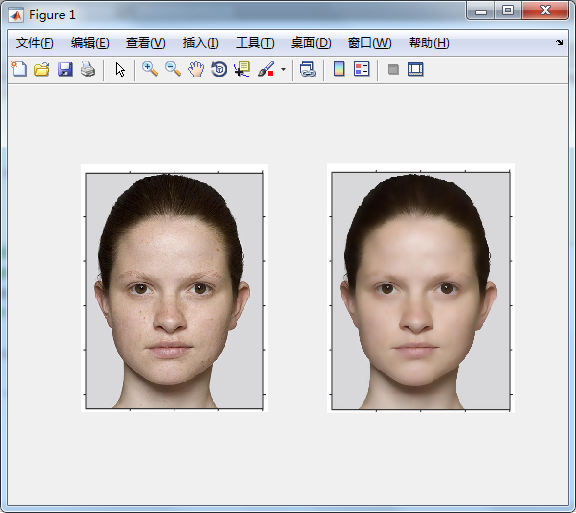展开全文双边滤波 matlab代码
• ## 【图像处理】——双边滤波

万次阅读 多人点赞 2018-11-26 12:33:39
{W_{q}}=\sum_{p\in S}G_{s}(p)G_{r}(p)=\sum_{p\in S}exp(-\frac{\left \| p-q \right \|^{2}}{2\sigma _{s}^{2}})exp(-\frac{\left \| I_{p}-I_{q} \right \|^{2}}{2\sigma _{r}^{2}}) W q ​ = p ∈ S ∑ ​ G ...
【fishing-pan：https://blog.csdn.net/u013921430 转载请注明出处】
双边滤波
高斯滤波是最常用的图像去噪方法之一，它能很好地滤除掉图像中随机出现的高斯噪声，但是在之前的博客中提到过，高斯滤波是一种低通滤波（有兴趣的点击这里，查看之前的博客），它在滤除图像中噪声信号的同时，也会对图像中的边缘信息进行平滑，表现出来的结果就是图像变得模糊，如下图所示；高斯滤波之所以会导致图像变得模糊，是因为它在滤波过程中只关注了位置信息；例如，以

q

q

为中心的窗口中，某一点

p

p

在高斯滤波过程中的权重的计算方法如下式；

G

(

p

)

=

1

2

π

σ

2

e

−

∥

p

−

q

∥

2

2

σ

2

G(p)=\frac{1}{2\pi \sigma ^{2}} e^{-\frac{\left \| p-q \right \|^{2}}{2\sigma ^{2}}}

即在滤波窗口内，距离中心点越近的点的权重越大；这种只关注距离的思想在某些情况下是可行的，例如在平坦的区域，距离越近的区域其像素分布也越相近，自然地，这些点的像素值对滤波中心点的像素值更有参考价值。但是在像素值出现跃变的边缘区域，这种方法会适得其反，损失掉有用的边缘信息。此时就出现了一类算法——边缘保护滤波方法，双边滤波就是最常用的边缘保护滤波方法（另一种常用来与双边滤波对比的边缘保护滤波方法——引导滤波）。
双边滤波的思想很简单，在高斯滤波的基础上加入了像素值权重项，也就是说既要考虑距离因素，也要考虑像素值差异的影响，像素值越相近，权重越大。将像素值权重表示为

G

r

G_{r}

，空间距离权重表示为

G

s

G_{s}

。

G

s

=

e

x

p

(

−

∥

p

−

q

∥

2

2

σ

s

2

)

G_{s}=exp(-\frac{\left \| p-q \right \|^{2}}{2\sigma _{s}^{2}})

G

r

=

e

x

p

(

−

∥

I

p

−

I

q

∥

2

2

σ

r

2

)

G_{r}=exp(-\frac{\left \| I_{p}-I_{q} \right \|^{2}}{2\sigma _{r}^{2}})

那么整个滤波器可以表示为

B

F

BF

，那么滤波结果为；

B

F

=

1

W

q

∑

p

∈

S

G

s

(

p

)

G

r

(

p

)

∗

I

p

=

1

W

q

∑

p

∈

S

e

x

p

(

−

∥

p

−

q

∥

2

2

σ

s

2

)

e

x

p

(

−

∥

I

p

−

I

q

∥

2

2

σ

r

2

)

∗

I

p

\begin{aligned} BF&amp;=\frac{1}{W_{q}}\sum_{p\in S}G_{s}(p)G_{r}(p)*I_{p}\\ &amp;=\frac{1}{W_{q}}\sum_{p\in S}exp(-\frac{\left \| p-q \right \|^{2}}{2\sigma _{s}^{2}})exp(-\frac{\left \| I_{p}-I_{q} \right \|^{2}}{2\sigma _{r}^{2}})*I_{p} \end{aligned}

其中

W

q

{W_{q}}

为滤波窗口内每个像素值的权重和，用于权重的归一化；

W

q

=

∑

p

∈

S

G

s

(

p

)

G

r

(

p

)

=

∑

p

∈

S

e

x

p

(

−

∥

p

−

q

∥

2

2

σ

s

2

)

e

x

p

(

−

∥

I

p

−

I

q

∥

2

2

σ

r

2

)

{W_{q}}=\sum_{p\in S}G_{s}(p)G_{r}(p)=\sum_{p\in S}exp(-\frac{\left \| p-q \right \|^{2}}{2\sigma _{s}^{2}})exp(-\frac{\left \| I_{p}-I_{q} \right \|^{2}}{2\sigma _{r}^{2}})

在平坦区域，滤波器中每个像素点的

G

r

G_{r}

值相近，空间距离权重

G

s

G_{s}

主导滤波效果。在边缘区域，边缘同侧的

G

r

G_{r}

值相近，且远大于边缘另一侧的

G

r

G_{r}

值，此时另一侧的像素点的权重对滤波结果几乎不影响，边缘信息得到保护。表现出了一定的自适应性。
在平坦区域出现噪声点时，噪声点周围的信号的权值都很小，在进行归一化后，这些权值得到了提升，因此对噪声点也有滤除效果。双边滤波OpenCV实现
在OpenCV中已经有编辑好的双边滤波的函数；
void bilateralFilter( InputArray src, OutputArray dst, int d,
double sigmaColor, double sigmaSpace,
int borderType = BORDER_DEFAULT );

函数中的参数依次表示src：输入图像，dst: 输出图像，d：滤波窗口的直径（函数注释中使用的是Diameter，那么很可能函数中选取的窗口是圆形窗口），sigmaColor：像素值域方差，sigmaSpace：空间域方差，以及边缘处理方式。
根据算法原理，我编写了自己的双边滤波函数；其中的核心部分如下；
//-------------------------------------------------------------
//作者：不用先生，2018.11.26
//自实现的图像双边滤波算法
//bilateral.cpp
//-------------------------------------------------------------
for (int i = 0; i < row; i++)    //对每一个点进行处理
{
for (int j = 0; j < col; j++)
{
double weightSum = 0;
double filterValue = 0;
for (int row_d = -(d / 2); row_d <= (d / 2); row_d++)   //以图像中的一点为中心，d为边长的方形区域内进行计算
{
for (int col_d = -(d / 2); col_d <= (d / 2); col_d++)
{
double distance_Square = row_d*row_d + col_d*col_d;
double value_Square = pow((scr.at<uchar>(i, j) - copyBorder_dst.at<uchar>(i + (d / 2) + row_d, j + (d / 2) + col_d)), 2);
double weight = exp(-1 * (distance_Square / (2 * sigmaSpace*sigmaSpace) + value_Square / (2 * sigmaColor*sigmaColor)));
weightSum += weight;               //求滤波窗口内的权重和，用于归一化；
filterValue += (weight*copyBorder_dst.at<uchar>(i + (d / 2) + row_d, j + (d / 2) + col_d));

}
}
dst.at<uchar>(i, j) = filterValue / weightSum;

}
}

从代码中可以看出，我选择的是一个方形区域而不是圆形区域进行滤波计算，大家如果觉得欠妥，在自己编写代码时可以修改成圆形区域，也很简单。完整代码，可以到这里下载，或者直接在评论区留下自己的邮箱，我看到留言后会尽快发的您的邮箱。
测试结果
用自己编写的代码、OpenCV自带的双边滤波函数以及高斯滤波函数分别对一张彩色图像今进行处理，并对比了结果。这里出现了很奇怪的现象，所有输入参数相同时（我选取参数的是d=21；sigmaColor=10；sigmaSpace = 10；），我的函数计算的结果比OpenCV自带的 bilateralFilter 函数的处理结果更加平滑。当把 bilateralFilter 函数的sigmaColor和sigmaSpace参数调整为两到三倍的时候， bilateralFilter 函数的结果与我的计算结果相近。这种差异性的结果不应该是窗口选取方式不同导致的。具体原因，我觉得可能与 bilateralFilter 函数中方差的应用有关。但是总的来说，两个函数都对图像有一定的平滑效果，并很好地保护了边缘信息。
本博客中只讲述了双边滤波最基础的原理以及实现过程，这种计算方法比较耗时，后续的人们做了一系列的工作，用于提升双边滤波的计算效率。大家感兴趣的可以去了解一下。
参考

Tomasi C, Manduchi R. Bilateral filtering for gray and color images[C]//Computer Vision, 1998. Sixth International Conference on. IEEE, 1998: 839-846.He K, Sun J, Tang X. Guided image filtering[J]. IEEE transactions on pattern analysis & machine intelligence, 2013 (6): 1397-1409.

已完。。
展开全文双边滤波 OpenCV实现
• 基于正交小波包的心电R波检测。signal是12导心电信号，R1是返回的R波波峰的位置。
• w(i,j,k,l) = d(i,j,k,l) * r(i,j,k,l) = exp(-\frac{(i-k)^2 + (j-l)^2}{2\sigma_d^2} - \frac{||f(i,j) - f(k,l)||^2}{2\sigma_r^2}) w ( i , j , k , l ) = d ( i , j , k , l ) ∗ r ( i , j , k , l ) = e x p ...快速双边滤波
• ## 双边滤波

千次阅读 2018-01-23 17:06:20
sigma_r = 100*sigma_r;    % Create waitbar.  h = waitbar(0, 'Applying bilateral filter...' );  set(h, 'Name' , 'Bilateral Filter Progress' );    % Apply bilateral ...
• 通过对心电信号进行八层平稳小波变换，得到不同的小系数，采用变量阈值函数表达式对其进行处理得到新的小系数，最后进行逆平稳小波变换实现小重构，完成心电信号去噪。Matlab 仿真结果显示，本文算法的准确...
• 采用氮化铌和铝作为制备微悬臂梁的材料，其中氮化铌用来吸收太赫兹辐射，铝作为形变材料。利用氮化铌和铝的热膨胀系数相差较大的特性以及在吸热后产生形变的特点，与材料微悬臂梁阵列探测红外技术相结合，...
• 图像滤波原理之双边滤波图像滤波问题描述1. 空间(即图像定义域)相邻关系的滤波器2....令I∈Rh×w×cI\in R^{h\times w\times c}I∈Rh×w×c表示图像是一个二维矩阵，h,wh,wh,w分别表示图像的高度和宽度，c=1,3c=1,3...图像处理 滤波 滤波原理 双边滤波
• 分析和小相关的实验代码（matlab），可用于长时间序列数据的变化周期特点分析和两个或者多个时间序列数据的相关性分析（文件夹里包含一份空气质量的实验数据，可用于参照）。
• ## 双边滤波实现-python

千次阅读 2020-07-23 22:21:42
其中，高斯滤波函数是： Gas[i,j]=e−(i2+j2)2∗σ2，−r,j<r,r表示核的半径Gas[i,j]=e^{\frac{-(i^2+j^2)}{2*\sigma^2}}，-r Gas[i,j]=e2∗σ2−(i2+j2)​，−r,j<r,r表示核的半径 对用的空间滤波器的 Spa[i,j]=e...
• w=r*d ），当前像素受到的影响就越小，从而保持了边缘的细节信息。 思想：抑制与中心像素值差异较大的像素（即使你们空域相距较近）。   计算方法：对每一个邻域像素点，计算出其对应的空域系数和值域系数，...双边滤波 OpenCV
• 第五节：图像平滑2 一：联合双边滤波 代码实现： import numpy as np import cv2 import math def getClosenessWeight(sigma_g, H, W): ... r, c = np.mgrid[0:H:1, 0:W:1] # 构造三维表 r -= int...opencv3 Python 联合双边滤波 计算机视觉
• ## 双边滤波——原理及matlab实现

万次阅读 多人点赞 2018-08-18 20:53:41
temp3 = fb_temp(i-r:i+r,j-r:j+r); dr = temp1 - fr_temp(i,j); dg = temp2 - fg_temp(i,j); db = temp3 - fb_temp(i,j); w2 = exp(-(dr.^2+dg.^2+db.^2)/(2*b^2)); w = w1.*w2; gr(i-r,j-r) = sum(sum(temp...
• 在非旋近似下对光子Jaynes-Cummings（J-C）模型与单模相干态光场相互作用的量子特性进行了精确求解。对光子J-C 模型与单光子J-C 模型量子纠缠和原子布居数反转的演化特点进行了对比，讨论了平均光子数、光场与...
• 高斯滤波函数、双边滤波函数的MATLAB实现高斯滤波函数双边滤波函数 自己编写滤波函数，剖析滤波内部结构 ... r=3; % 高斯模板的参数 NoiseI= imnoise(Image,'gaussian'); % 加噪,添加高斯噪声 gaus...
• ## 双边滤波原理（Bilateral Filtering）

万次阅读 多人点赞 2017-12-18 22:49:15
sigma_r 表示值域的差别，因此强调这一差别，即减小 sigma_r 可以突出边缘。 sigma_d 变大，图像每个区域的权重基本都源于值域滤波的权重，因此对于空间邻域信息不是很敏感；sigma_r 变大，则不太考虑值域，...
• double weight_tmp = d_metrix[m + r][n + r] * r_metrix[pixcel_dif]; // 复合权重 pixcel_sum += img_tmp[y_tmp * m_nChannels * nx + m_nChannels * x_tmp + k] * weight_tmp; weight_sum += ...图像处理 双边滤波 c++
• Mask2.at(r + hh, c + ww) = colorWeight2 * spaceMask.at(r + hh, c + ww); space_color_sum = space_color_sum + Mask0.at(r + hh, c + ww); space_color_sum = space_color_sum + ...
• 双边滤波与一般的高斯滤波的不同就是：双边滤波既利用了位置信息几何信息——高斯滤波...C Tomasi, R Manduchi. Bilateral Filtering for Gray and Color Images,  - Computer Vision, 1998.图像处理
• 如图1.1.1（1）所示，三个窄脉冲形状不同，但是它们的面积都等于1，当它们分别加在如图1.1.1（2）（a）所示的R-L电路上时，并设其电流i（t）为电路的输出，则其输出响应波形基本相同且如图1.1.1（2）（b）所示。...
• 我们表明，对应于F1 / pp对偶对的场理论（DFT）的状解决方案更恰当地是DFT作用与σ模型作用耦合的解决方案。 产生pp弦配置可以认为是静态量规，且弦指向双向。 我们还将讨论与振动弦相对应的DFT解决...
• 在现有彩色图像水印提取技术基础上，提出一种结合9/7正交提升小和图像矩阵奇异值分解的彩色图像水印加密算法。首先将彩色原始图像和彩色水印图像R、G、B分离，将图像相应分量进行提升小三级分解得到低频部分，...矩阵奇异值分解 水印加密 Arnold置乱
• double gaussian_r_coeff = -0.5 / (sigma_r * sigma_r); double **d_metrix = new double *[w_filter]; for (int i = 0; i ; ++i) d_metrix[i] = new double[w_filter]; double r_metrix; // ...opencv c++ 图像处理
• 1. 滤波器介绍 滤波器作为图像处理课程的重要内容，大致可分为两类，空域滤波器和频率域滤波器。本文主要介绍常用的四种滤波器：中值滤波器、均值滤波器、高斯滤波器、双边滤波器，并基于opencv做出实现。...
• 下双边滤波里的两个权重域的概念：空间域（spatial domain S）和像素范围域（range domain R）,这个是它跟高斯滤波等方法的最大不同点。下面是我找到的对比说明，更好地理解双边滤波，首先是高斯滤波的情况： ...双边滤波
• 心电信号QRS复实时检测,软件主要是检测出R波波峰位置，采用阈值法进行检测。配有GUI演示界面。所用数据为MIT，1986年心电数据库。 此为医学信号处理实验课程作业，与大家分享
• ## PCL双边滤波

千次阅读 2016-11-16 14:32:28
bf.setStdDev(sigma_r);   bf.filter(outcloud);       // 保存滤波输出点云文件   pcl::io::savePCDFile(outcloudfile.c_str(), outcloud);   return (0);  }  ...
• R>95 && G>40 && B>20 && R>G && R>B && Max(R,G,B)- Min(R,G,B)>15 Abs(R-G)>15  另外还有其他基于 YUV ， YCbC 等色彩空间的肤色检测，但都效果不佳（包括RGB， 例图：尽可能阐释算法的优点的图片）。 ...高斯滤波 双边滤波 肤色检测...